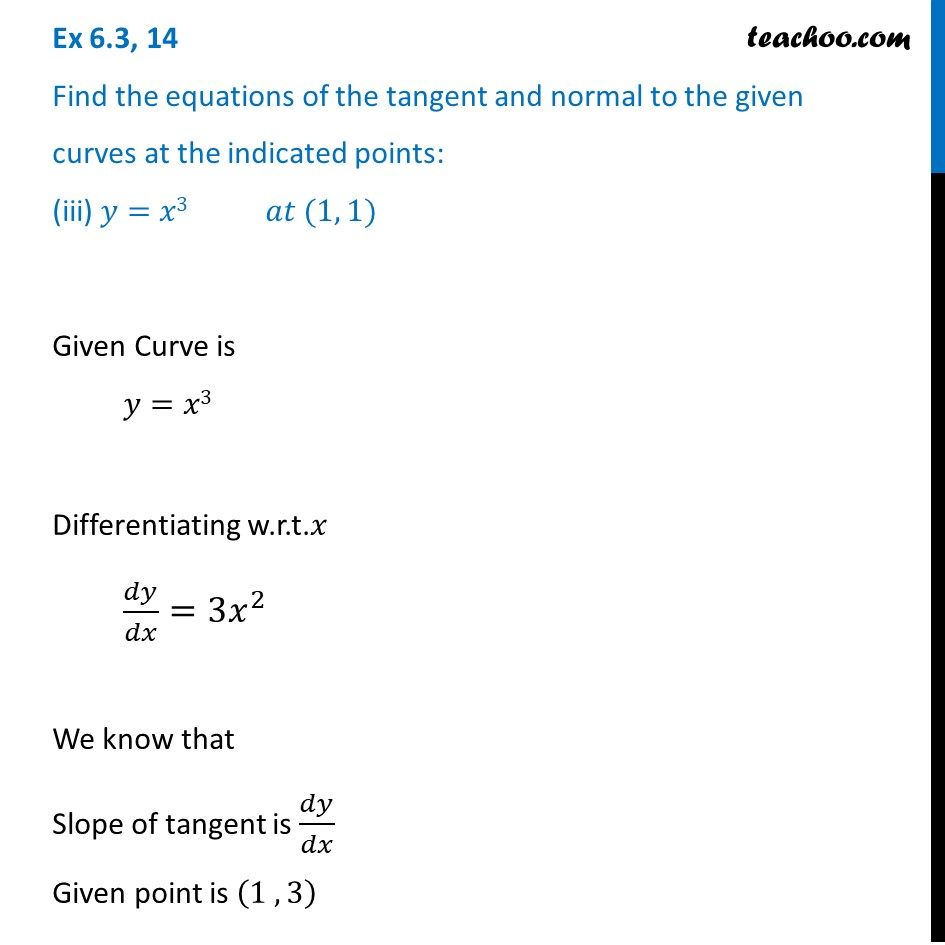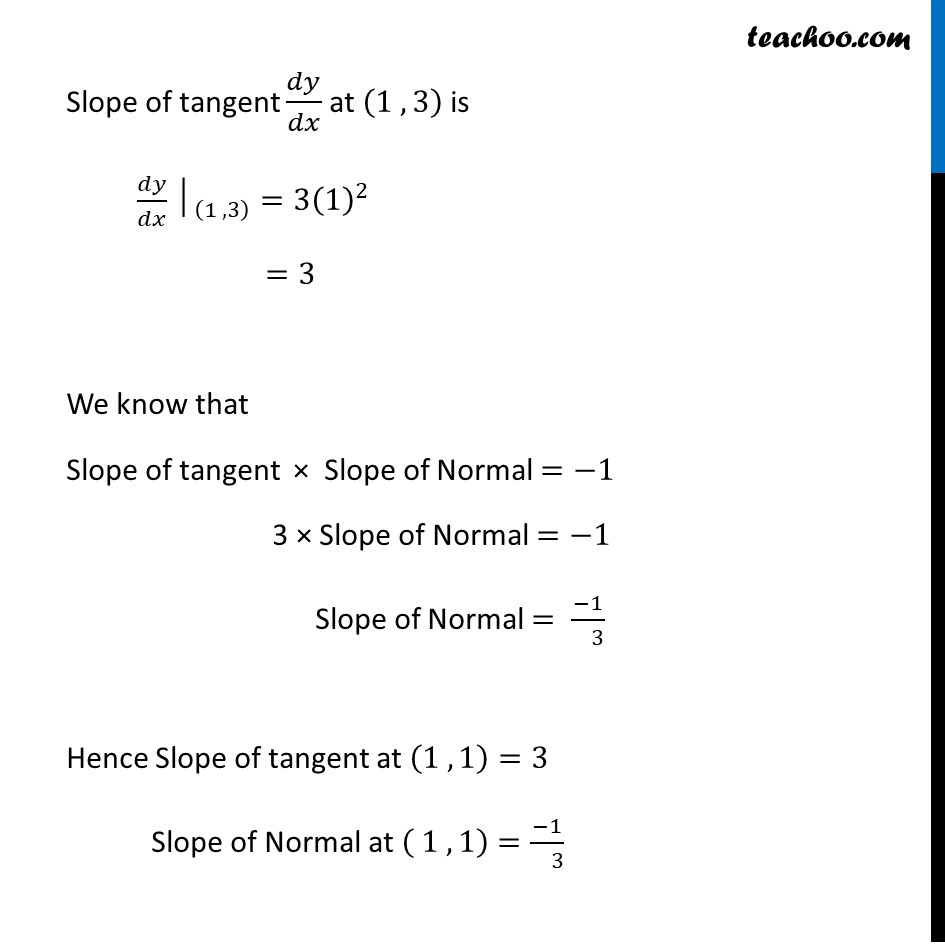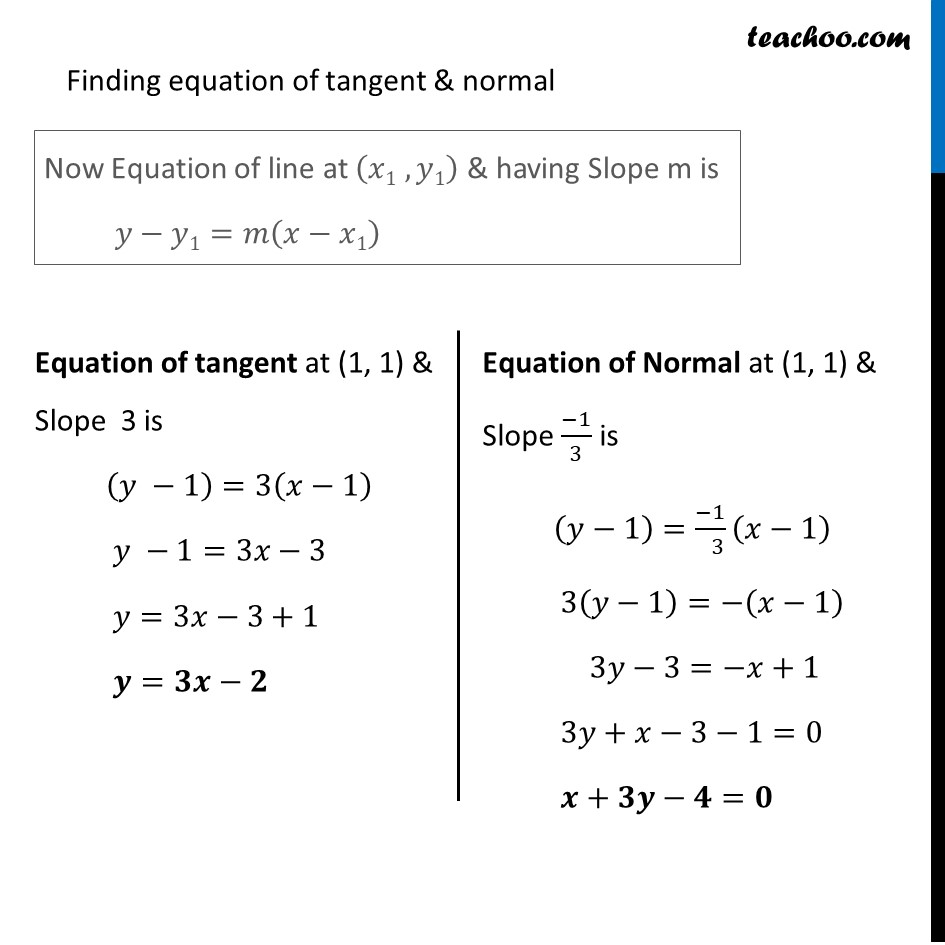Tangents and Normals (using Differentiation)

Chapter 6 Class 12 Application of Derivatives
Serial order wiseLearn in your speed, with individual attention - Teachoo Maths 1-on-1 Class

### Transcript

Question 14 Find the equations of the tangent and normal to the given curves at the indicated points: (iii) 𝑦=𝑥3 𝑎𝑡 (1, 1)Given Curve is 𝑦=𝑥3 Differentiating w.r.t.𝑥 𝑑𝑦/𝑑𝑥=3𝑥^2 We know that Slope of tangent is 𝑑𝑦/𝑑𝑥 Given point is (1 , 3) Slope of tangent 𝑑𝑦/𝑑𝑥 at (1 , 3) is 〖𝑑𝑦/𝑑𝑥│〗_((1 , 3) )=3(1)^2 =3 We know that Slope of tangent × Slope of Normal =−1 3 × Slope of Normal =−1 Slope of Normal = (−1)/( 3) Hence Slope of tangent at (1 , 1)=3 Slope of Normal at ( 1 , 1)=(−1)/( 3) Finding equation of tangent & normal Now Equation of line at (𝑥1 , 𝑦1) & having Slope m is 𝑦−𝑦1=𝑚(𝑥−𝑥1) Equation of tangent at (1, 1) & Slope 3 is (𝑦 −1)=3(𝑥−1) 𝑦 −1=3𝑥−3 𝑦=3𝑥−3+1 𝒚=𝟑𝒙−𝟐 Equation of Normal at (1, 1) & Slope (−1)/3 is (𝑦−1)=(−1)/( 3) (𝑥−1) 3(𝑦−1)=−(𝑥−1) 3𝑦−3=−𝑥+1 3𝑦+𝑥−3−1=0 𝒙+𝟑𝒚−𝟒=𝟎